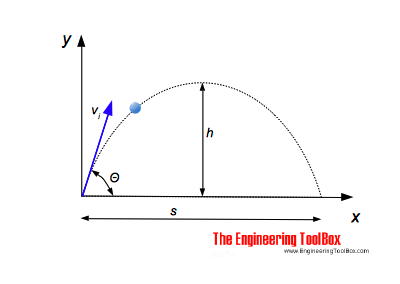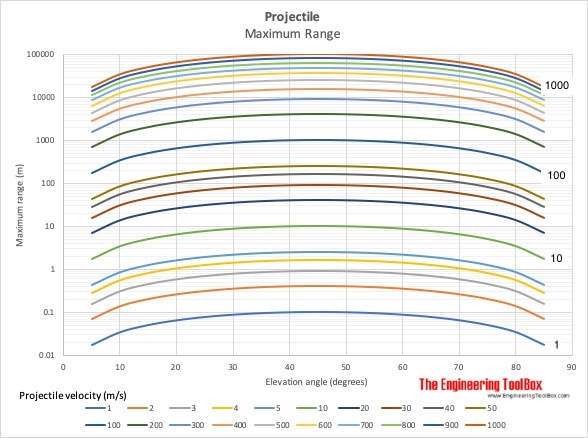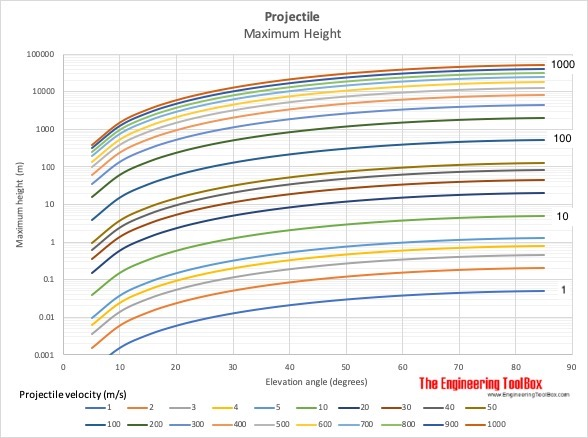Engineering ToolBox - Resources, Tools and Basic Information for Engineering and Design of Technical Applications!

# Projectile Range

## Calculate the range of a projectile - a motion in two dimensions.The time for a projectile - a bullet, a ball or a stone or something similar - thrown out with an angle Θ to the horizontal plane - to reach the maximum height can be calculated as

th = vi sin(Θ) / ag                                 (1)

where

th = time to reach maximum height (s)

vi = initial velocity of the projectile (m/s, ft/s)

Θ = the initial angle of the velocity vector to the horizontal plane (degrees)

ag = acceleration of gravity (9.81 m/s232.174 ft/s2)

The total flight time can be expressed as

ts = 2 th

= 2 vi sin(Θ) / ag                      (2)

where

ts = time for the total flight (s)

The maximum elevation - h - of the flight can be calculated  as

h = 1/2 ag th2                              (3)

where

h = flight maximum elevation (m, ft)

The horizontal distance of the flight can be expressed as

s = vi2 sin(2 Θ) / ag                           (4)

where

s = flight distance (m, ft)

### Example - Throwing a Ball

A ball is thrown with initial velocity 25 m/s in angle 30 degrees to the horizontal plane. The time for the ball to reach maximum level is

th = (25 m/s) sin(30 degrees) / (9.81 m/s2)

= 1.27

The maximum elevation of the ball can be calculated as

h = 1/2 (9.81 m/s2) (1.27 s)2

= 7.91 m

The horizontal distance traveled by the ball can be calculated as

s = (25 m/s)2 sin(2 30 degrees) / (9.81 m/s2)

= 55.2 m

### Projectile Flight Calculator

The calculator below can be used to estimate the time of flight, time to reach maximum elevation, maximum elevation and flight distance for a projectile like a bullet or a ball or something similar.

Note that friction force due to air resistance is neglected.

vi - initial velocity (m/s)

Θ - initial angle to the horizontal plane (degrees)Note that friction force due to air resistance is neglected in the charts above. For higher velocities range and maximum height will be dramatically reduced.

## Related Topics

• Mechanics - Forces, acceleration, displacement, vectors, motion, momentum, energy of objects and more.
• Dynamics - Motion - velocity and acceleration, forces and torque.

## Engineering ToolBox - SketchUp Extension - Online 3D modeling!

Add standard and customized parametric components - like flange beams, lumbers, piping, stairs and more - to your Sketchup model with the Engineering ToolBox - SketchUp Extension - enabled for use with the amazing, fun and free SketchUp Make and SketchUp Pro .Add the Engineering ToolBox extension to your SketchUp from the SketchUp Pro Sketchup Extension Warehouse!

Translate

## Privacy

We don't collect information from our users. Only emails and answers are saved in our archive. Cookies are only used in the browser to improve user experience.

Some of our calculators and applications let you save application data to your local computer. These applications will - due to browser restrictions - send data between your browser and our server. We don't save this data.

## Citation

• Engineering ToolBox, (2014). Projectile Range. [online] Available at: https://www.engineeringtoolbox.com/range-projectile-d_1908.html [Accessed Day Mo. Year].

Modify access date.

. .

#### Scientific Online Calculator3 30